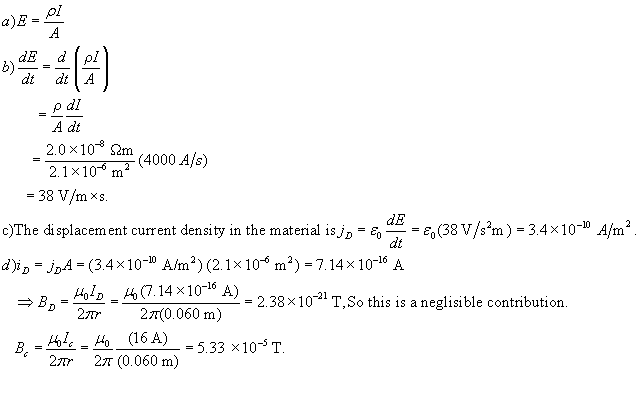# A long, straight, copper wire with a circular cross-sectional area of 2.1mm^2 carries a current of 16 A. The resistivity of the material is 2.0\times1beljuA 2021-03-30 Answered
A long, straight, copper wire with a circular cross-sectional area of $2.1m{m}^{2}$ carries a current of 16 A. The resistivity of the material is $2.0×{10}^{-8}$ Om.
a) What is the uniform electric field in the material?
b) If the current is changing at the rate of 4000 A/s, at whatrate is the electric field in the material changing?
c) What is the displacement current density in the material in part (b)?
d) If the current is changing as in part (b), what is the magnitude of the magnetic field 6.0cm from the center of the wire? Note that both the conduction current and the displacement currentshould be included in the calculation of B. Is the contribution from the displacement current significant?
You can still ask an expert for help

• Questions are typically answered in as fast as 30 minutes

Solve your problem for the price of one coffee

• Math expert for every subject
• Pay only if we can solve ittimbalemX
I provide the solution below:###### Not exactly what you’re looking for?Jeffrey Jordon

a) $E=\frac{\rho l}{A}$

b) $\frac{dE}{dt}=\frac{d}{dt}\left(\frac{\rho l}{A}\right)$

$=\frac{\rho }{A}\frac{dl}{dt}$

c) The dispacement current in the material is

d)

$⇒{B}_{p}=\frac{{\mu }_{0}{I}_{p}}{2\pi r}=\frac{{\mu }_{0}\left(7.14×{10}^{-16}A\right)}{2\pi \left(0.060m\right)}=2.38×{10}^{-21}T$ So this is a neglisible contribution